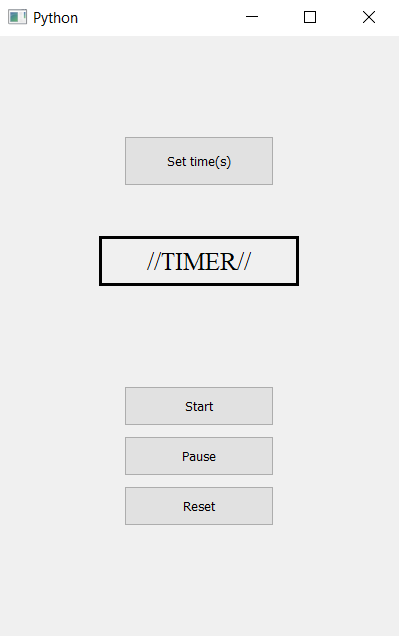# Timer Application using PyQt5

In this article we will see how we can create a timer application in PyQt5. A timer is a specialized type of clock used for measuring specific time intervals, for the given time we have to decrease the time until times become zero i.e counting downwards.GUI Implementation steps :

1. Create a push button to open pop up for getting time and set its geometry
2. Create label to show time and complete status
3. Set label geometry, font size and align its text to center
4. Create three push button for starting the timer, pausing the timer and for resetting the timer
5. Set the geometry of each button

Back-end Implementation steps :

1. Create count variable and flag to know if counter is stopped or running
2. Add action to each button
3. Inside the get second button action get the value of second using input dialog box and make the flag false
4. Inside the start action make flag true but if count is zero make if false
5. Inside pause action make flag false
6. Inside the reset action make flag false, set count value to zero and set text to the label
7. Make a timer object which calls its method after every 100 milliseconds
8. Inside the timer action check for the flag then decrement the value of count and set text to the label

Below is the implementation

 `# importing libraries ` `from` `PyQt5.QtWidgets ``import` `*`  `from` `PyQt5 ``import` `QtCore, QtGui ` `from` `PyQt5.QtGui ``import` `*`  `from` `PyQt5.QtCore ``import` `*`  `import` `sys ` ` `  ` `  `class` `Window(QMainWindow): ` ` `  `    ``def` `__init__(``self``): ` `        ``super``().__init__() ` ` `  `        ``# setting title ` `        ``self``.setWindowTitle(``"Python "``) ` ` `  `        ``# setting geometry ` `        ``self``.setGeometry(``100``, ``100``, ``400``, ``600``) ` ` `  `        ``# calling method ` `        ``self``.UiComponents() ` ` `  `        ``# showing all the widgets ` `        ``self``.show() ` ` `  `    ``# method for widgets ` `    ``def` `UiComponents(``self``): ` ` `  `        ``# variables ` `        ``# count variable ` `        ``self``.count ``=` `0` ` `  `        ``# start flag ` `        ``self``.start ``=` `False` ` `  `        ``# creating push button to get time in seconds ` `        ``button ``=` `QPushButton(``"Set time(s)"``, ``self``) ` ` `  `        ``# setting geometry to the push button ` `        ``button.setGeometry(``125``, ``100``, ``150``, ``50``) ` ` `  `        ``# adding action to the button ` `        ``button.clicked.connect(``self``.get_seconds) ` ` `  `        ``# creating label to show the seconds ` `        ``self``.label ``=` `QLabel(``"//TIMER//"``, ``self``) ` ` `  `        ``# setting geometry of label ` `        ``self``.label.setGeometry(``100``, ``200``, ``200``, ``50``) ` ` `  `        ``# setting border to the label ` `        ``self``.label.setStyleSheet(``"border : 3px solid black"``) ` ` `  `        ``# setting font to the label ` `        ``self``.label.setFont(QFont(``'Times'``, ``15``)) ` ` `  `        ``# setting alignment ot the label ` `        ``self``.label.setAlignment(Qt.AlignCenter) ` ` `  `        ``# creating start button ` `        ``start_button ``=` `QPushButton(``"Start"``, ``self``) ` ` `  `        ``# setting geometry to the button ` `        ``start_button.setGeometry(``125``, ``350``, ``150``, ``40``) ` ` `  `        ``# adding action to the button ` `        ``start_button.clicked.connect(``self``.start_action) ` ` `  `        ``# creating pause button ` `        ``pause_button ``=` `QPushButton(``"Pause"``, ``self``) ` ` `  `        ``# setting geometry to the button ` `        ``pause_button.setGeometry(``125``, ``400``, ``150``, ``40``) ` ` `  `        ``# adding action to the button ` `        ``pause_button.clicked.connect(``self``.pause_action) ` ` `  `        ``# creating reset  button ` `        ``reset_button ``=` `QPushButton(``"Reset"``, ``self``) ` ` `  `        ``# setting geometry to the button ` `        ``reset_button.setGeometry(``125``, ``450``, ``150``, ``40``) ` ` `  `        ``# adding action to the button ` `        ``reset_button.clicked.connect(``self``.reset_action) ` ` `  `        ``# creating a timer object ` `        ``timer ``=` `QTimer(``self``) ` ` `  `        ``# adding action to timer ` `        ``timer.timeout.connect(``self``.showTime) ` ` `  `        ``# update the timer every tenth second ` `        ``timer.start(``100``) ` ` `  `    ``# method called by timer ` `    ``def` `showTime(``self``): ` ` `  `        ``# checking if flag is true ` `        ``if` `self``.start: ` `            ``# incrementing the counter ` `            ``self``.count ``-``=` `1` ` `  `            ``# timer is completed ` `            ``if` `self``.count ``=``=` `0``: ` ` `  `                ``# making flag false ` `                ``self``.start ``=` `False` ` `  `                ``# setting text to the label ` `                ``self``.label.setText(``"Completed !!!! "``) ` ` `  `        ``if` `self``.start: ` `            ``# getting text from count ` `            ``text ``=` `str``(``self``.count ``/` `10``) ``+` `" s"` ` `  `            ``# showing text ` `            ``self``.label.setText(text) ` ` `  ` `  `    ``# method called by the push button ` `    ``def` `get_seconds(``self``): ` ` `  `        ``# making flag false ` `        ``self``.start ``=` `False` ` `  `        ``# getting seconds and flag ` `        ``second, done ``=` `QInputDialog.getInt(``self``, ``'Seconds'``, ``'Enter Seconds:'``) ` ` `  `        ``# if flag is true ` `        ``if` `done: ` `            ``# changing the value of count ` `            ``self``.count ``=` `second ``*` `10` ` `  `            ``# setting text to the label ` `            ``self``.label.setText(``str``(second)) ` ` `  `    ``def` `start_action(``self``): ` `        ``# making flag true ` `        ``self``.start ``=` `True` ` `  `        ``# count = 0 ` `        ``if` `self``.count ``=``=` `0``: ` `            ``self``.start ``=` `False` ` `  `    ``def` `pause_action(``self``): ` ` `  `        ``# making flag false ` `        ``self``.start ``=` `False` ` `  `    ``def` `reset_action(``self``): ` ` `  `        ``# making flag false ` `        ``self``.start ``=` `False` ` `  `        ``# setting count value to 0 ` `        ``self``.count ``=` `0` ` `  `        ``# setting label text ` `        ``self``.label.setText(``"//TIMER//"``) ` ` `  ` `  ` `  `# create pyqt5 app ` `App ``=` `QApplication(sys.argv) ` ` `  `# create the instance of our Window ` `window ``=` `Window() ` ` `  `# start the app ` `sys.exit(App.``exec``()) `

Output :

My Personal Notes arrow_drop_upCheck out this Author's contributed articles.

If you like GeeksforGeeks and would like to contribute, you can also write an article using contribute.geeksforgeeks.org or mail your article to contribute@geeksforgeeks.org. See your article appearing on the GeeksforGeeks main page and help other Geeks.

Please Improve this article if you find anything incorrect by clicking on the "Improve Article" button below.

Article Tags :

Be the First to upvote.

Please write to us at contribute@geeksforgeeks.org to report any issue with the above content.